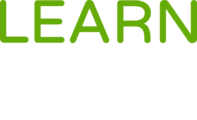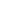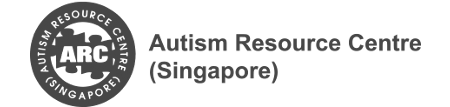#The Mole is a Course

## The Mole

Started 5 Feb 2020

### Full course description

This course is a basic introduction to "The Mole", a basic unit of measurement used in stoichiometry (the quantitative study of chemicals).

This course contains three modules:

• Atoms and Relative Atomic Mass of Elements
• Relative Molecular Mass and Relative Formula Mass
• What Is a Mole, Avogadro’s Constant and Chemical Formula

Students will learn to:

• define relative atomic mass (Ar)
• define relative molecular mass (Mr)
• calculate relative molecular mass (and relative formula mass) as the sum of relative atomic masses
• calculate the number of moles of substances in a reaction.
• calculate the volume of a gas at room temperature and pressure.
• calculate the concentration of solutions in g/dm3 or mol/dm3

This online course is open for Pathlight School students to access.

Pathlight School students can enrol in this course after logging in to Learn for Life eCampus.## Chemistry

Pathlight School

### Offered Byacademics, academic-bridging, science, chemistry, mole, stoichiometry, symbols-of-elements, formulae-of-compounds, state-symbols, chemical-formulae, chemical-equations, ionic-equations, relative-atomic-mass, relative-atomic-mass, empirical-formulae, molecular-formulae, secondary, upper-secondary, intermediate,